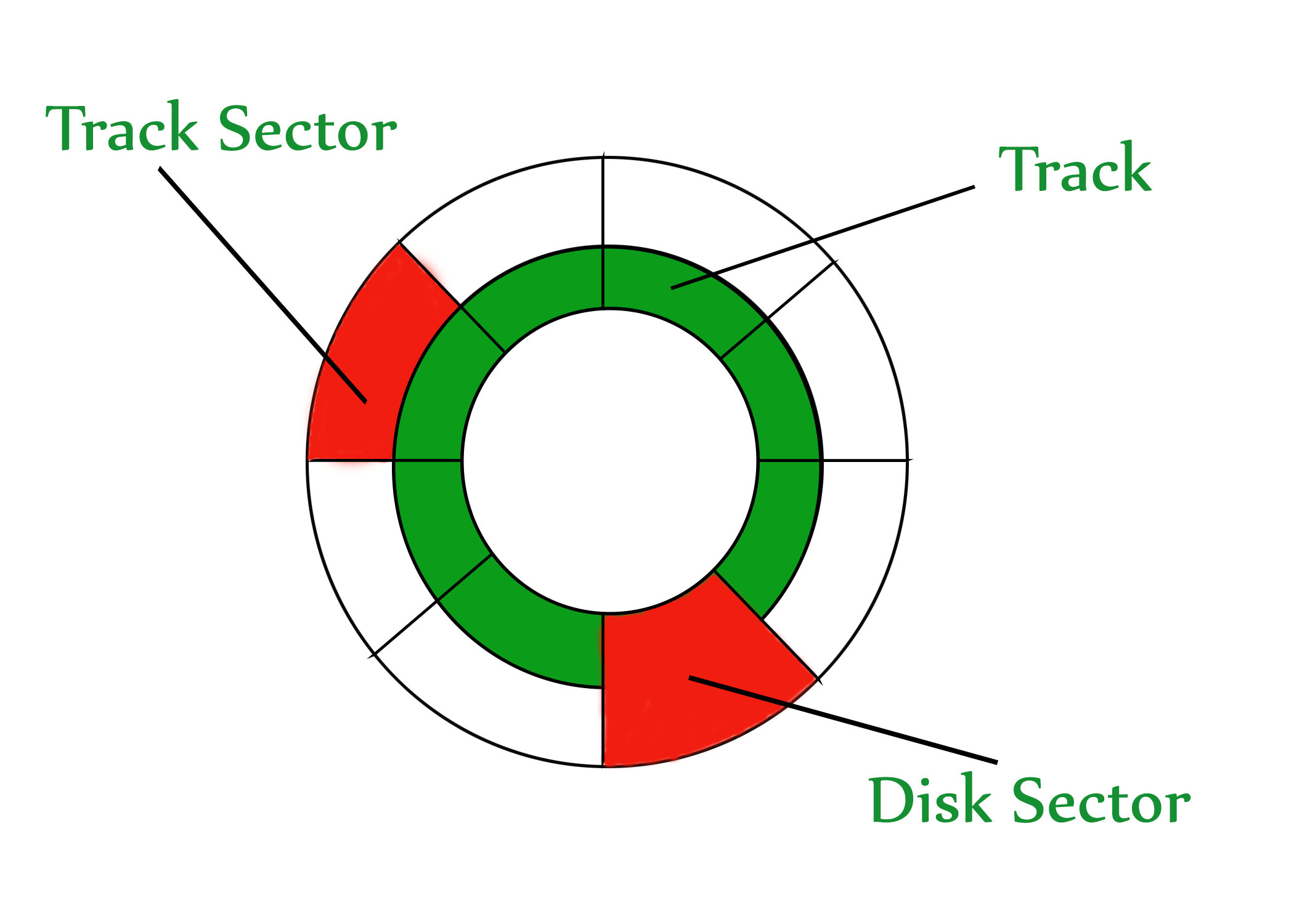Open in App
Not now

# Hard Disk Drive (HDD) Secondary memory

• Difficulty Level : Easy
• Last Updated : 24 Jan, 2022

A hard disk is a memory storage device that looks like this:The disk is divided into tracks. Each track is further divided into sectors. The point to be noted here is that outer tracks are bigger in size than the inner tracks but they contain the same number of sectors and have equal storage capacity. This is because the storage density is high in sectors of the inner tracks whereas the bits are sparsely arranged in sectors of the outer tracks. Some space of every sector is used for formatting. So, the actual capacity of a sector is less than the given capacity.

Read-Write(R-W) head moves over the rotating hard disk. It is this Read-Write head that performs all the read and writes operations on the disk and hence, the position of the R-W head is a major concern. To perform a read or write operation on a memory location, we need to place the R-W head over that position. Some important terms must be noted here:

1. Seek time – The time taken by the R-W head to reach the desired track from its current position.
2. Rotational latency – Time is taken by the sector to come under the R-W head.
3. Data transfer time – Time is taken to transfer the required amount of data. It depends upon the rotational speed.
4. Controller time – The processing time taken by the controller.
5. Average Access time – seek time + Average Rotational latency + data transfer time + controller time.

Note: Average Rotational latency is mostly 1/2*(Rotational latency).

In questions, if the seek time and controller time are not mentioned, take them to be zero.

If the amount of data to be transferred is not given, assume that no data is being transferred. Otherwise, calculate the time taken to transfer the given amount of data.

The average rotational latency is taken when the current position of the R-W head is not given. Because the R-W may be already present at the desired position or it might take a whole rotation to get the desired sector under the R-W head. But, if the current position of the R-W head is given then the rotational latency must be calculated.

Example –
Consider a hard disk with:
4 surfaces
64 tracks/surface
128 sectors/track
256 bytes/sector

1. What is the capacity of the hard disk?
Disk capacity = surfaces * tracks/surface * sectors/track * bytes/sector
Disk capacity = 4 * 64 * 128 * 256
Disk capacity = 8 MB
2. The disk is rotating at 3600 RPM, what is the data transfer rate?
60 sec -> 3600 rotations
1 sec -> 60 rotations
Data transfer rate = number of rotations per second * track capacity * number of surfaces (since 1 R-W head is used for each surface)
Data transfer rate = 60 * 128 * 256 * 4
Data transfer rate = 7.5 MB/sec
3. The disk is rotating at 3600 RPM, what is the average access time?
Since seek time, controller time and the amount of data to be transferred is not given, we consider all three terms as 0.
Therefore, Average Access time = Average rotational delay
Rotational latency => 60 sec -> 3600 rotations
1 sec -> 60 rotations
Rotational latency = (1/60) sec = 16.67 msec.
Average Rotational latency = (16.67)/2
= 8.33 msec.
Average Access time = 8.33 msec.
4. Another example: GATE IT 2007 | Question 44

My Personal Notes arrow_drop_up
Related Articles